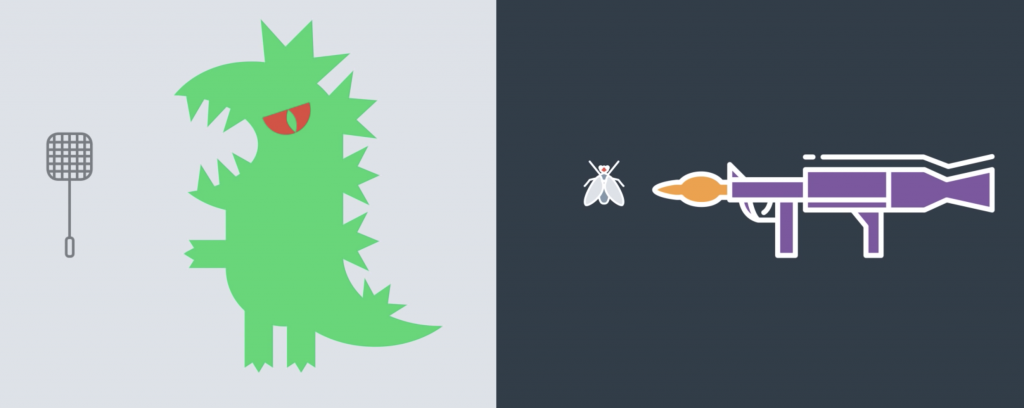# Avoid overfitting using cross-validation

Original article was published by Larawehbe on Becoming Human: Artificial Intelligence MagazineFolding Validation sets using Cross-Validation!

1 — Overfitting in Transfer learning

2 — Avoiding overfitting using k-fold cross-validation

3 — Coding part

Transfer Learning is a term that has crossed the field of deep learning lately and been used so far.

A quick recall about transfer learning: Using pre-trained models to train yours in case you don’t have enough dataset for the new dataset.

A Kaggle competition caught my attention weeks ago that I felt intrigued to give it a try. It was centered on image classification and the candidate is up to choose one of two topics: heart disease and grocery items.

So, I chose the grocery items. They were 19 classes with significantly very low data!

For this reason, using Transfer learning is a must. But, a problem that anyone will encounter is overfitting. Overfitting can be simply put as the following:

The model can recognize the training dataset too well but lacks the ability to learn the dataset features, so it fails to predict new unseen data.

At first, the loss that the model produced was very high and the accuracy didn’t go above 0.1!. Analyzing the graphs produced to realize that it is an overfitting major problem. Hence, K-Fold Cross-validation was the best choice.

K-Fold Cross-Validation

Simply speaking, it is an algorithm that helps to divide the training dataset into k parts(folds). Within each epoch, (k-1) folds will be taken as training data, and the last part will be a testing part for predictions. The latter part is called the “holdout fold”. Each time, the holdout fold will change. A kind of shuffle k-times will take place within the k-parts.

How will this affect the model and alleviate the overfitting?

Actually, the problem with overfitting is that the model gets ‘over-familiar’ with the training data. To avoid such a scenario, we will use cross-validation.

### Trending AI Articles:

1. Fundamentals of AI, ML and Deep Learning for Product Managers

2. The Unfortunate Power of Deep Learning

3. Graph Neural Network for 3D Object Detection in a Point Cloud

4. Know the biggest Notable difference between AI vs. Machine Learning

Coding part || Fun part

The built-in K-fold function is found in sklearn library.

`'''X : is the training dataset train_index: is the index of of the training set same for the test index'''`
`for train_index,test_index in KFold(n_split).split(X):              `
`    x_train,x_test=X[train_index],X[test_index]    y_train,y_test=Y[train_index],Y[test_index]    model=create_model()`

The code above does the following :

• iterates overall training and testing objects in the k fold.
• creates a new training set and a new testing set
• calls the create_model function to create the model and find the output

BUT, where is the create_model() function? WE WILL CREATE IT NOW 🙂

create_model function ( description of each line below the code)

`def create_model():`
`   IMAGE_SIZE = [100, 100] #fixed image size`
`   vgg = VGG16(input_shape=IMAGE_SIZE + , weights='imagenet',     include_top=False) #get the weigths of imagenet that are used in vgg#include top = false is to get all the layers of vgg except the one that takes the specific features of the model`
`   for layer in vgg.layers:`
`      layer.trainable = False #dont train the layers of vgg, because we need their weights fixed`
`   y1 = Flatten()(vgg.output)`
`   bn2 = BatchNormalization()(y1)`
`   y4 = Dense(37, activation='relu')(bn2)`
`   bn3 = BatchNormalization()(y1)`
`   prediction = Dense(3, activation='softmax')(bn3)`
`   model = Model(inputs=vgg.input, outputs=prediction)`
`   model.compile(loss='categorical_crossentropy',`
` optimizer=optimizers.Adam(),`
`   metrics=['accuracy'])`
`   model.summary()   return model  `
• Since we are dealing with the transfer learning image classification model, we decided to choose VGG16 for its ability to memorize and analyze small features in the images.
• The image size is set to (100,100) for convenience between most of the images.
• ‘include_top = false’ is a primary condition in transfer learning, it is the ability to get the most benefit of the trained model, and putting all trained weights in the needed model ( current one).
• After that, we don’t want the VGG16 to train again from the beginning, because the weights must be frozen from the vgg. In this case, we will loop over all trainable layers and make them false.
• Since the model is now ready, let’s start adding the top layer that was excluded from the last layer :

1 — Flatten(): that flattens all the outputs

2 — BatchNormalization(): normalizes the output of the layer to make them all convenient which helps the model to understand the results more ( all results between 0 and 1 is much better than that between 0 and 1000! )

3 — Dense(37,activation=’relu’) : why 37 units ? actually, it is a tough process that demands a lot of try and error to find out the best unit number for the hidden layers. At first, I started with 22 and found the worst results ever. Then, I tried 97 and found the same except in an opposite direction. So, I started going down 10 units, then 5, then 1, until I got this!

( Note that each model needs its specific hidden units number so make sure you go through try and error )

4 — One more BatchNormalization

5 — The final Dense that will use activation function as ‘softmax’ in order to find out the probability of each class studied before, 3 units because I was trying to train the model on 3 classes for results observation only.

Finally, let’s check out the results :

#### Training without K-fold cross-validation :

Test Loss: 2.23648738861084

Test accuracy: 0.36900368332862854

#### Training with K-fold cross-validation : ( k = 5)

Test Loss: 0.9668004512786865

Test accuracy: 0.6000000238418579

In conclusion, the cross-validation approach has proved that it is the best fit to avoid overfitting!

### Don’t forget to give us your 👏 !Avoid overfitting using cross-validation was originally published in Becoming Human: Artificial Intelligence Magazine on Medium, where people are continuing the conversation by highlighting and responding to this story.